# Prepayment Modeling with a Two Factor Hull White Model and a LIBOR Market Model

This example shows how to model prepayment in MATLAB® using functionality from the Financial Instruments Toolbox™. Specifically, a variation of the Richard and Roll prepayment model is implemented using a two factor Hull-White interest-rate model and a LIBOR Market Model to simulate future interest-rate paths. A mortgage-backed security is priced with both the custom and default prepayment models.

### Introduction

Prepayment modeling is crucial to the analysis of mortgage-backed securities (MBS). Prepayments by individual mortgage holders affect both the amount and timing of cash flows and for collateralized mortgage obligations (for example, interest-only securities), prepayment can greatly affect the value of the securities.

### PSA Model

The most basic prepayment model is the Public Securities Association (PSA) model, which assumes a ramp-up phase and then a constant conditional prepayment rate (CPR). The PSA model can be generated in MATLAB using the Financial Instruments Toolbox function `psaspeed2rate`.

```G2PP_CPR = psaspeed2rate([100 200]); figure plot(G2PP_CPR) title('100 and 200 PSA Prepayment Speeds') xlabel('Months') ylabel('CPR') ylim([0 .14]) legend({'100 PSA','200 PSA'}, 'Location', 'Best')```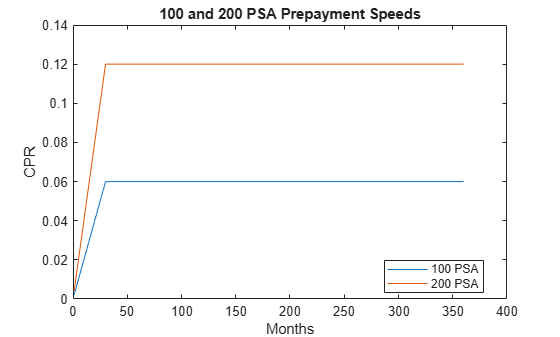### Mortgage-Backed Security

The MBS analyzed in this example matures in 2020 and has the properties outlined in this section. Cash flows are generated for PSA prepayment speeds simply by entering the PSA speed as an input argument.

```% Parameters for MBS passthrough to be priced Settle = datetime(2007,12,15); Maturity = datetime(2020,12,15); IssueDate = datetime(2000,12,15); GrossRate = .0475; CouponRate = .045; Delay = 14; Period = 12; Basis = 4; % Generate cash flows and dates for baseline case using 100 PSA [CFlowAmounts, CFlowDates] = mbscfamounts(Settle,Maturity, IssueDate,... GrossRate, CouponRate, Delay,100); CFlowTimes = yearfrac(Settle,CFlowDates); NumCouponsRemaining = cpncount(Settle, Maturity, Period,Basis, 1, IssueDate);```

### Richard and Roll Model

While prepayment modeling often involves complex and sophisticated modeling, often at the loan level, this example uses a slightly modified approach based on the model proposed by Richard and Roll .

The Richard and Roll prepayment model involves the following factors:

• Refinancing incentive

• Seasonality (month of the year)

• Seasoning or age of the mortgage

• Burnout

Richard and Roll propose a multiplicative model of the following:

`$CPR=RefiIncentive*SeasoningMultiplier*SeasonalityMultiplier*BurnoutMultiplier$`

For the custom model in this example, the Burnout Multiplier, which describes the tendency of prepayment to slow when a significant number of homeowners have already refinanced, is ignored and the first three terms are used.

The refinancing incentive is a function of the ratio of the coupon-rate of the mortgage to the available mortgage rate at that particular point in time. For example, the Office of Thrift Supervision (OTS) proposes the following model:

`$Refi=.2406-.1389*arctan\left(5.952*\left(1.089-\frac{CouponRate}{MortgageRate}\right)\right)$`

The refinancing incentive requires a simulation of future interest rates. This will be discussed later in this example.

```C_M = .1:.1:2; G2PP_Refi = .2406 - .1389 * atan(5.952*(1.089 - C_M)); figure plot(C_M,G2PP_Refi) xlabel('Coupon/Mortgage Rate') ylabel('CPR') title('Refinancing Incentive')```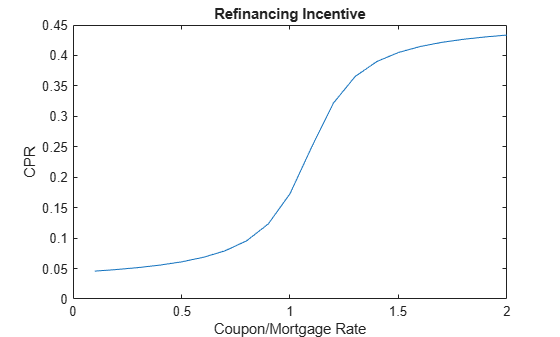Seasoning captures the tendency of prepayment to ramp up at the beginning of a mortgage before leveling off. The OTS models the seasoning multiplier as follows:

```Seasoning = ones(360,1); Seasoning(1:29) = (1:29)/30; figure plot(Seasoning) xlim([1 360]) title('Seasoning Multiplier') xlabel('Months')```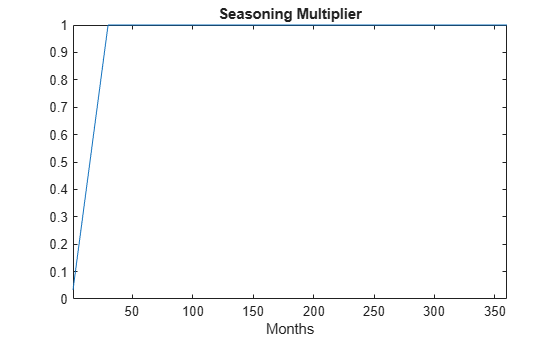The seasonality multiplier simply models the seasonal behavior of prepayments -- this data is based on Figure 3 of , which applies to the behavior of Ginnie Mae 30-year, single-family MBSs.

```Seasonality = [.94 .76 .73 .96 .98 .92 .99 1.1 1.18 1.21 1.23 .97]; figure plot(Seasonality) xlim([1 12]) ax = gca; ax.XTick = 1:12; ax.XTickLabel = {'Jan','Feb','Mar','Apr','May','Jun','Jul','Aug', ... 'Sep','Oct','Nov','Dec'}; title('Seasonality Multiplier')```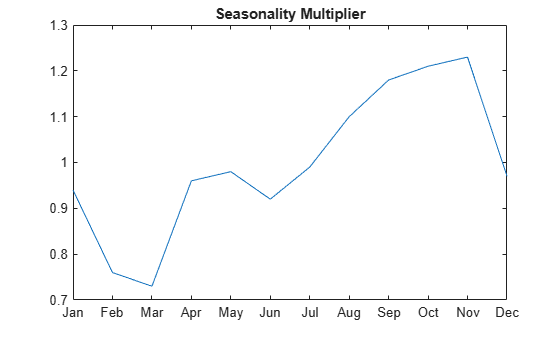### G2++ Interest-Rate Model

Since the refinancing incentive requires a simulation of future interest rates, an interest-rate model must be used. One choice is a two-factor additive Gaussian model, referred to as G2++ by Brigo and Mercurio .

The G2++ Interest Rate Model is:

`$r\left(t\right)=x\left(t\right)+y\left(t\right)+\phi \left(t\right)$`

`$dx\left(t\right)=-ax\left(t\right)dt+\sigma d{W}_{1}\left(t\right)$`

`$dy\left(t\right)=-by\left(t\right)dt+\eta d{W}_{2}\left(t\right)$`

where $d{W}_{1}\left(t\right)d{W}_{2}\left(t\right)$ is a two-dimensional Brownian motion with correlation $\rho$

`$d{W}_{1}\left(t\right)d{W}_{2}\left(t\right)=\rho dt$`

`$\phi \left(T\right)={f}^{M}\left(0,T\right)+\frac{{\sigma }^{2}}{2{a}^{2}}\left(1-{e}^{-aT}{\right)}^{2}+\frac{{\eta }^{2}}{2{b}^{2}}\left(1-{e}^{-bT}{\right)}^{2}+\rho \frac{\sigma \eta }{ab}\left(1-{e}^{-aT}\right)\left(1-{e}^{-bT}\right)$`

and $r\left(t\right)$ is the short rate, $a$ and $b$ are mean reversion constants and $\sigma$ and $\eta$ are volatility constants, and ${f}^{M}\left(0,T\right)$ is the market forward rate, or the forward rate observed on the Settle date.

### LIBOR Market Model

The LIBOR Market Model (LMM) differs from short-rate models in that it evolves a set of discrete forward rates. Specifically, the lognormal LMM specifies the following diffusion equation for each forward rate:

`$\frac{d{F}_{i}\left(t\right)}{{F}_{i}}=-{\mu }_{i}dt+{\sigma }_{i}\left(t\right)d{W}_{i}$`

where

dW is an N dimensional geometric Brownian motion with:

`$d{W}_{i}\left(t\right)d{W}_{j}\left(t\right)={\rho }_{ij}dt$`

The LMM relates the drifts of the forward rates based on no-arbitrage arguments. Specifically, under the Spot LIBOR measure, the drifts are expressed as the following:

`${\mu }_{i}\left(t\right)=-{\sigma }_{i}\left(t\right)\sum _{j=q\left(t\right)}^{i}\frac{{\tau }_{j}{\rho }_{i,j}{\sigma }_{j}\left(t\right){F}_{j}\left(t\right)}{1+{\tau }_{j}{F}_{j}\left(t\right)}$`

where

${\tau }_{i}$ is the time fraction associated with the ith forward rate

$q\left(t\right)$ is an index function defined by the relation ${T}_{q\left(t\right)-1}

and the Spot LIBOR numeraire is defined as the following:

`$B\left(t\right)=P\left(t,{T}_{q\left(t\right)}\right)\prod _{n=0}^{q\left(t\right)-1}\left(1+{\tau }_{n}{F}_{n}\left({T}_{n}\right)\right)$`

Given the above, the choice with the LMM is how to model volatility and correlation.

The volatility of the rates can be modeled with a stochastic volatility, but for this example a deterministic volatility is used, and so a functional form needs to be specified. One of the most popular functional forms in the literature is the following:

`${\sigma }_{i}\left(t\right)={\varphi }_{i}\left(a\left({T}_{i}-t\right)+b\right){e}^{c\left({T}_{i}-t\right)}+d$`

where $\varphi$ adjusts the curve to match the volatility for the ${i}^{th}$ forward rate.

Similarly, the correlation between the forward rates needs to be specified. This can be estimated from historical data or fitted to option prices. For this example, the following functional form will be used:

`${\rho }_{i,j}={e}^{-\beta |i-j|}$`

Once the volatility and correlation are specified, the parameters need to be calibrated -- this can be done with historical or market data, typically swaptions or caps and floors. For this example, we simply use reasonable estimates for the correlation and volatility parameters.

```% The volatility function to be used -- and one choice for the parameters LMMVolFunc = @(a,t) (a(1)*t + a(2)).*exp(-a(3)*t) + a(4); LMMVolParams = [.13 .04 .7 .08]; % Volatility specification fplot(@(t) LMMVolFunc(LMMVolParams,t),[0 10]) title(['Volatility Function with parameters ' mat2str(LMMVolParams)]) ylabel('Volatility (%)') xlabel('Tenor (years)')```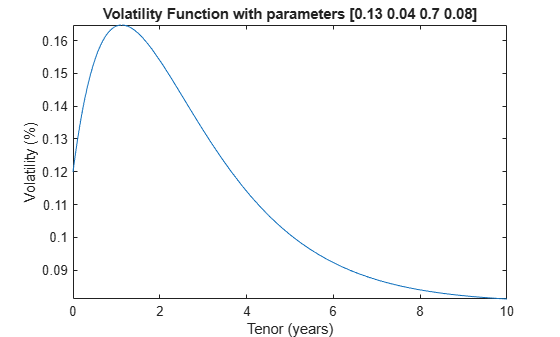### Calibration to Market Data

The parameters in the G2++ model can be calibrated to market data. Typically, the parameters are calibrated to observed interest-rate cap, floor and/or swaption data. For now, market cap data is used for calibration.

This data is hardcoded but could be imported into MATLAB with the Database Toolbox™ or Datafeed Toolbox™.

```% Zero Curve -- this data is hardcoded for now, but could be bootstrapped % using the bootstrap method of IRDataCurve. ZeroTimes = [3/12 6/12 1 5 7 10 20 30]'; ZeroRates = [0.033 0.034 0.035 0.040 0.042 0.044 0.048 0.0475]'; ZeroDates = daysadd(Settle,360*ZeroTimes,1); DiscountRates = zero2disc(ZeroRates,ZeroDates,Settle); irdc = IRDataCurve('Zero',Settle,ZeroDates,ZeroRates); figure plot(ZeroDates,ZeroRates) datetick title(['US Zero Curve for ' datestr(Settle)])```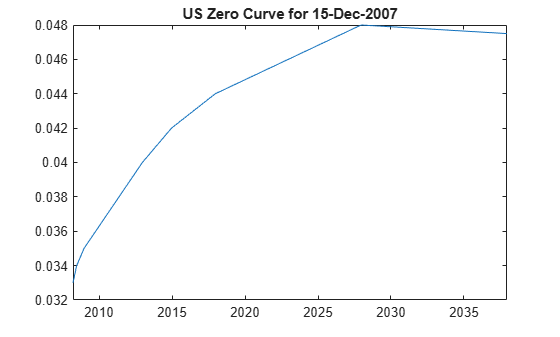```% Cap Data Reset = 2; Notional = 100; CapMaturity = daysadd(Settle,360*[1:5 7 10 15 20 25 30],1); CapVolatility = [.28 .30 .32 .31 .30 .27 .23 .2 .18 .17 .165]'; % ATM strikes could be computed with swapbyzero Strike = [0.0353 0.0366 0.0378 0.0390 0.0402 0.0421 0.0439 ... 0.0456 0.0471 0.0471 0.0471]'; % This could be computed with capbyblk BlackCapPrices = [0.1532 0.6416 1.3366 2.0290 2.7366 4.2960 6.5992 ... 9.6787 12.2580 14.0969 15.7873]'; figure scatter(CapMaturity,CapVolatility) datetick title(['ATM Volatility for Caps on ' datestr(Settle)])```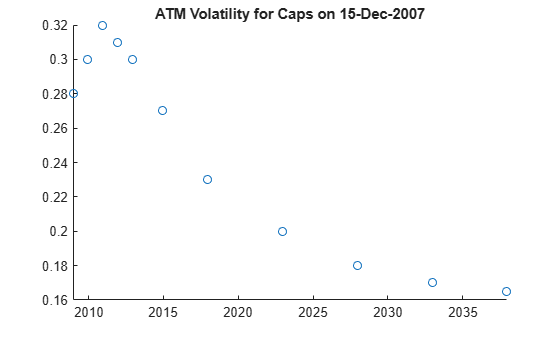To calibrate the model parameters, a parameter set will be found that minimizes the sum of the squared differences between the G2++ predicted Cap values and the observed Black Cap values. The Optimization Toolbox™ function `lsqnonlin` is used in this example, although other approaches (for example, Global Optimization) may also be applicable. The function `capbylg2f` computes the analytic values for the caps given parameter values.

Upper and lower bounds for the model parameters are set to be relatively constrained. As Brigo and Mercurio discuss, the correlation parameter, $rho$, can often be close to `-1` when fitting a G2++ model to interest-rate cap prices. Therefore, $rho$ is constrained to be between `-.7` and `.7` to ensure that the parameters represent a truly two-factor model. The remaining mean reversion and volatility parameters are constrained to be between `0` and `.5`. Calibration remains a complex task, and while the plot below indicates that the best fit parameters seem to do a reasonably good job of reproducing the Cap prices, it should be noted that the procedure outlined here simply represents one approach.

```% Call to lsqnonlin to calibrate parameters objfun = @(x) BlackCapPrices - capbylg2f(irdc,x(1),x(2),x(3),x(4),x(5),Strike,CapMaturity); x0 = [.5 .05 .1 .01 -.1]; lb = [0 0 0 0 -.7]; ub = [.5 .5 .5 .5 .7]; G2PP_Params = lsqnonlin(objfun,x0,lb,ub);```
```Local minimum possible. lsqnonlin stopped because the final change in the sum of squares relative to its initial value is less than the value of the function tolerance. ```
```a = G2PP_Params(1); b = G2PP_Params(2); sigma = G2PP_Params(3); eta = G2PP_Params(4); rho = G2PP_Params(5); % Compare the results figure scatter(CapMaturity,BlackCapPrices) hold on scatter(CapMaturity,capbylg2f(irdc,a,b,sigma,eta,rho,Strike,CapMaturity),'rx') datetick title('Market and Model Implied Prices') ylabel('Price (\$)')```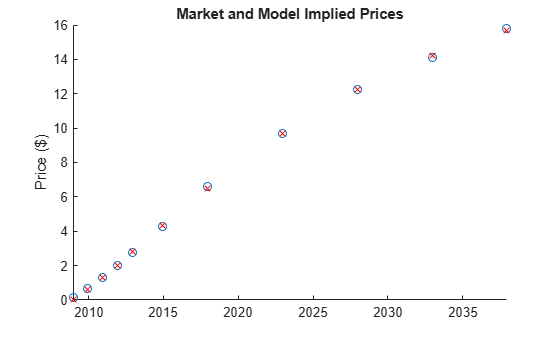### G2++ Model Implementation

The `LinearGaussian2F` model can be used to specify the G2++ model and simulate future paths interest rates.

```% G2++ model from Brigo and Mercurio with time homogeneous volatility % parameters G2PP = LinearGaussian2F(irdc,a,b,sigma,eta,rho);```

### LIBOR Market Model Implementation

After the volatility and correlation have been calibrated, Monte Carlo simulation is used to evolve the rates forward in time. The `LiborMarketModel` object is used to simulate the forward rates.

While factor reduction is often used with the LMM to reduce computational complexity, there is no factor reduction in this example.

6M LIBOR rates are chosen to be evolved in this simulation. Since a monthly prepayment vector must be computed, interpolation is used to generate the intermediate rates. Simple linear interpolation is used.

```numForwardRates = 46; % Instead of being fit, VolPhi is simply hard-coded -- % representative of a declining volatility over time. VolPhi = linspace(1.2,.8,numForwardRates-1)'; Beta = .08; CorrFunc = @(i,j,Beta) exp(-Beta*abs(i-j)); CorrMat = CorrFunc(meshgrid(1:numForwardRates-1)',meshgrid(1:numForwardRates-1),Beta); VolFunc = cell(length(VolPhi),1); for jdx = 1:length(VolPhi) VolFunc(jdx) = {@(t) VolPhi(jdx)*ones(size(t)).*(LMMVolParams(1)*t + ... LMMVolParams(2)).*exp(-LMMVolParams(3)*t) + LMMVolParams(4)}; end LMM = LiborMarketModel(irdc,VolFunc,CorrMat);```

### G2++ Monte Carlo Simulation

The various interest-rate paths can be simulated by calling the `simTermStructs` method.

One limitation to two-factor Gaussian models like this one is that it does permit negative interest rates. This is a concern, particularly in low interest-rate environments. To handle this possibility, any interest-rate paths with negative rates are simply rejected.

```nPeriods = NumCouponsRemaining; nTrials = 100; DeltaTime = 1/12; % Generate factors and short rates Tenor = [1/12 1 2 3 4 5 7 10 15 20 30]; G2PP_SimZeroRates = G2PP.simTermStructs(nPeriods,'NTRIALS',nTrials,... 'Tenor',Tenor,'DeltaTime',DeltaTime); SimDates = daysadd(Settle,360*DeltaTime*(0:nPeriods),1); % Tenors that will be recovered for each simulation date. The stepsize is % included here to facilitate computing a discount factor for each % simulation path. % Remove any paths that go negative NegIdx = squeeze(any(any(G2PP_SimZeroRates < 0,1),2)); G2PP_SimZeroRates(:,:,NegIdx) = []; nTrials = size(G2PP_SimZeroRates,3); % Plot evolution of one sample path trialIdx = 1; figure surf(Tenor,SimDates,G2PP_SimZeroRates(:,:,trialIdx)) datetick y keepticks keeplimits title(['Evolution of the Zero Curve for Trial:' num2str(trialIdx) ' of G2++ Model']) xlabel('Tenor (Years)')```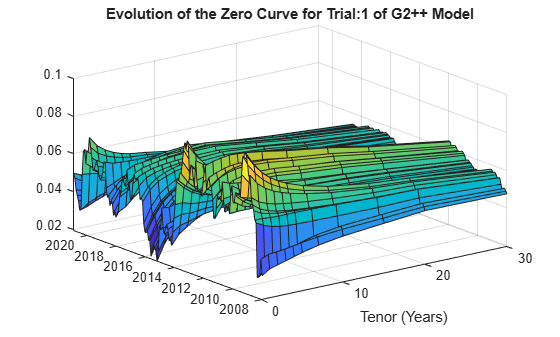### LIBOR Market Model Simulation

The various interest-rate paths can be simulated by calling the `simTermStructs` method of the `LiborMarketModel` object.

```LMMPeriod = 2; % Semiannual rates LMMNumPeriods = NumCouponsRemaining/12*LMMPeriod; % Number of semiannual periods LMMDeltaTime = 1/LMMPeriod; LMMNTRIALS = 100; % Simulate [LMMZeroRates, LMMForwardRates] = LMM.simTermStructs(LMMNumPeriods,'nTrials',LMMNTRIALS,'DeltaTime',LMMDeltaTime); ForwardTimes = 1/2:1/2:numForwardRates/2; LMMSimTimes = 0:1/LMMPeriod:LMMNumPeriods/LMMPeriod; % Plot evolution of one sample path trialIdx = 1; figure tmpPlotData = LMMZeroRates(:,:,trialIdx); tmpPlotData(tmpPlotData == 0) = NaN; surf(ForwardTimes,LMMSimTimes,tmpPlotData) title(['Evolution of the Zero Curve for Trial:' num2str(trialIdx) ' of LIBOR Market Model']) xlabel('Tenor (Years)')```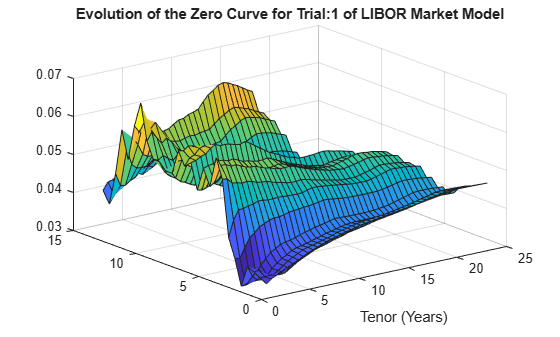### Compute Mortgage Rates from Simulation

Once the interest-rate paths have been simulated, the mortgage rate needs to be computed -- one approach, discussed by , is to compute the mortgage rate from a combination of the 2-year and 10-year rates.

For this example, the following is used:

`$MortgageRate=.024+.2*TwoYearRate+.6*TenYearRate$`

```% Compute mortgage rates from interest rate paths TwoYearRates = squeeze(G2PP_SimZeroRates(:,Tenor == 2,:)); TenYearRates = squeeze(G2PP_SimZeroRates(:,Tenor == 7,:)); G2PP_MortgageRates = .024 + .2*TwoYearRates + .6*TenYearRates; LMMMortgageRates = squeeze(.024 + .2*LMMZeroRates(:,4,:) + .6*LMMZeroRates(:,20,:)); LMMDiscountFactors = squeeze(cumprod(1./(1 + LMMZeroRates(:,1,:)*.5))); % Interpolate to get monthly mortgage rates MonthlySimTimes = 0:1/12:LMMNumPeriods/LMMPeriod; LMMMonthlyMortgageRates = zeros(nPeriods+1,LMMNTRIALS); LMMMonthlyDF = zeros(nPeriods+1,LMMNTRIALS); for trialidx = 1:LMMNTRIALS LMMMonthlyMortgageRates(:,trialidx) = interp1(LMMSimTimes,LMMMortgageRates(:,trialidx),MonthlySimTimes,'linear','extrap'); LMMMonthlyDF(:,trialidx) = interp1(LMMSimTimes,LMMDiscountFactors(:,trialidx),MonthlySimTimes,'linear','extrap'); end```

### Computing CPR and Generating and Valuing Cash Flows

Once the Mortgage Rates have been simulated, the CPR can be computed from the multiplicative model for each interest-rate path.

```% Compute Seasoning and Refinancing Multipliers Seasoning = ones(nPeriods+1,1); Seasoning(1:30) = 1/30*(1:30); G2PP_Refi = .2406 - .1389 * atan(5.952*(1.089 - CouponRate./G2PP_MortgageRates)); LMM_Refi = .2406 - .1389 * atan(5.952*(1.089 - CouponRate./LMMMonthlyMortgageRates)); % CPR is simply computed by evaluating the multiplicative model G2PP_CPR = bsxfun(@times,G2PP_Refi,Seasoning.*(Seasonality(month(CFlowDates))')); LMM_CPR = bsxfun(@times,LMM_Refi,Seasoning.*(Seasonality(month(CFlowDates))')); % Compute single monthly mortality (SMM) from CPR G2PP_SMM = 1 - (1 - G2PP_CPR).^(1/12); LMM_SMM = 1 - (1 - LMM_CPR).^(1/12); % Plot CPR's against 100 PSA CPR_PSA100 = psaspeed2rate(100); figure PSA_handle = plot(CPR_PSA100(1:nPeriods),'rx'); hold on G2PP_handle = plot(G2PP_CPR,'b'); LMM_handle = plot(LMM_CPR,'g'); title('Prepayment Speeds') legend([PSA_handle(1) G2PP_handle(1) LMM_handle(1)],{'100 PSA','G2PP CPR','LMM CPR'},'Location', 'Best');```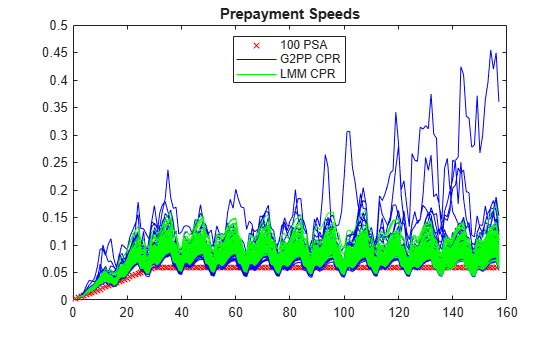### Generate Cash Flows and Compute Present Value

With a vector of single monthly mortalities (SMM) computed for each interest-rate path, cash flows for the MBS can be computed and discounted.

```% Compute the baseline zero rate at each cash flow time CFlowZero = interp1(ZeroTimes,ZeroRates,CFlowTimes,'linear','extrap'); % Compute DF for each cash flow time CFlowDF_Zero = zero2disc(CFlowZero,CFlowDates,Settle); % Compute the price of the MBS using the zero curve Price_Zero = CFlowAmounts*CFlowDF_Zero'; % Generate the cash flows for each IR Path G2PP_CFlowAmounts = mbscfamounts(Settle, ... repmat(Maturity,1,nTrials), IssueDate, GrossRate, CouponRate, Delay, [], G2PP_SMM(2:end,:)); % Compute the DF for each IR path G2PP_CFlowDFSim = cumprod(exp(squeeze(-G2PP_SimZeroRates(:,1,:).*DeltaTime))); % Present value the cash flows for each MBS G2PP_Price_Ind = sum(G2PP_CFlowAmounts.*G2PP_CFlowDFSim',2); G2PP_Price = mean(G2PP_Price_Ind); % Repeat for LMM LMM_CFlowAmounts = mbscfamounts(Settle, ... repmat(Maturity,1,LMMNTRIALS), IssueDate, GrossRate, CouponRate, Delay, [], LMM_SMM(2:end,:)); % Present value the cash flows for each MBS LMM_Price_Ind = sum(LMM_CFlowAmounts.*LMMMonthlyDF',2); LMM_Price = mean(LMM_Price_Ind);```

The results from the different approaches can be compared. The number of trials for the G2++ model will typically be less than 100 due to the filtering out of any paths that produce negative interest rates.

Additionally, while the number of trials for the G2++ model in this example is set to be 100, it is often the case that a larger number of simulations need to be run to produce an accurate valuation.

`fprintf(' # of Monte Carlo Trials: %8d\n' , nTrials)`
``` # of Monte Carlo Trials: 72 ```
`fprintf(' # of Time Periods/Trial: %8d\n\n' , nPeriods)`
``` # of Time Periods/Trial: 156 ```
`fprintf(' MBS Price with PSA 100: %8.4f\n' , Price_Zero)`
``` MBS Price with PSA 100: 1.0187 ```
`fprintf(' MBS Price with Custom G2PP Prepayment Model: %8.4f\n\n', G2PP_Price)`
``` MBS Price with Custom G2PP Prepayment Model: 0.9884 ```
`fprintf(' MBS Price with Custom LMM Prepayment Model: %8.4f\n\n', LMM_Price)`
``` MBS Price with Custom LMM Prepayment Model: 0.9993 ```

### Conclusion

This example shows how to calibrate and simulate a G2++ interest-rate model and how to use the generated interest-rate paths in a prepayment model loosely based on the Richard and Roll model. This example also provides a useful starting point to using the G2++ and LMM interest-rate models in other financial applications.

### Bibliography

This example is based on the following books, papers, and journal articles:

1. Andersen, L. and V. Piterbarg. Interest Rate Modeling. Atlantic Financial Press, 2010.

2. Brigo, D. and F. Mercurio. Interest Rate Models - Theory and Practice with Smile, Inflation and Credit (2nd ed. 2006 ed.). Springer Verlag. ISBN 978-3-540-22149-4, 2001.

3. Hayre, L., ed., Salomon Smith Barney Guide to Mortgage-Backed and Asset-Backed Securities. New York: John Wiley & Sons, 2001.

4. Karpishpan, Y., O. Turel, and A. Hasha. "Introducing the Citi LMM Term Structure Model for Mortgages". The Journal of Fixed Income. Volume 20. 44-58, 2010.

5. Rebonato, R., K. McKay, and R. White (2010). The Sabr/Libor Market Model: Pricing, Calibration and Hedging for Complex Interest-Rate Derivatives. John Wiley & Sons, 2010.

6. Richard, S. F., and R. Roll. "Prepayments on Fixed Rate Mortgage-Backed Securities". Journal of Portfolio Management. 1989.

7. Office of Thrift Supervision, "Net Portfolio Value Model Manual". March 2000.

8. Stein, H. J., Belikoff, A. L., Levin, K. and Tian, X. "Analysis of Mortgage Backed Securities: Before and after the Credit Crisis" (January 5, 2007). "Credit Risk Frontiers: Subprime Crisis, Pricing and Hedging, CVA, MBS, Ratings, and Liquidity". Bielecki, Tomasz,; Damiano Brigo and Frederic Patras., eds., February 2011. Available at SSRN: https://papers.ssrn.com/sol3/papers.cfm?abstract_id=955358